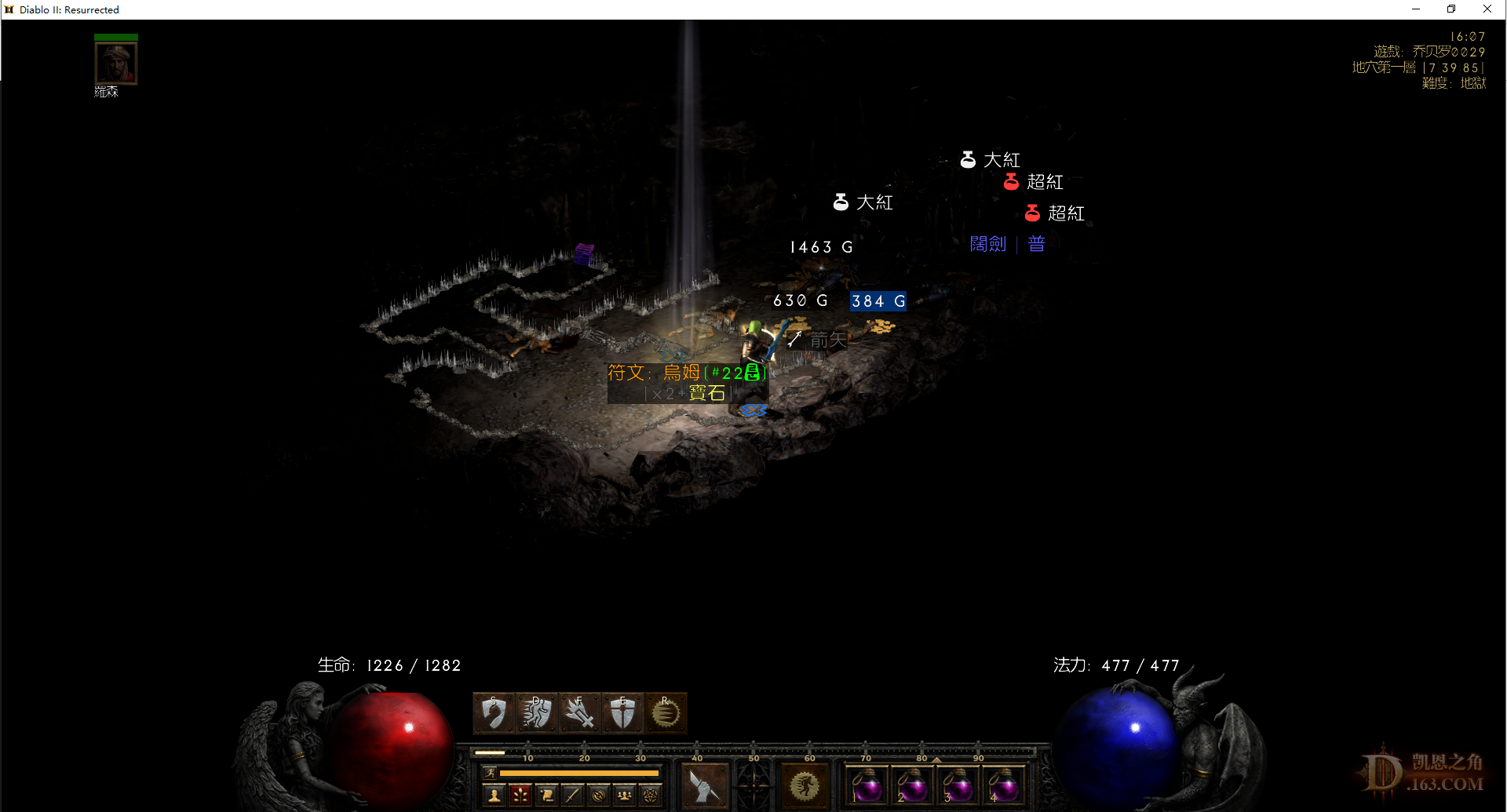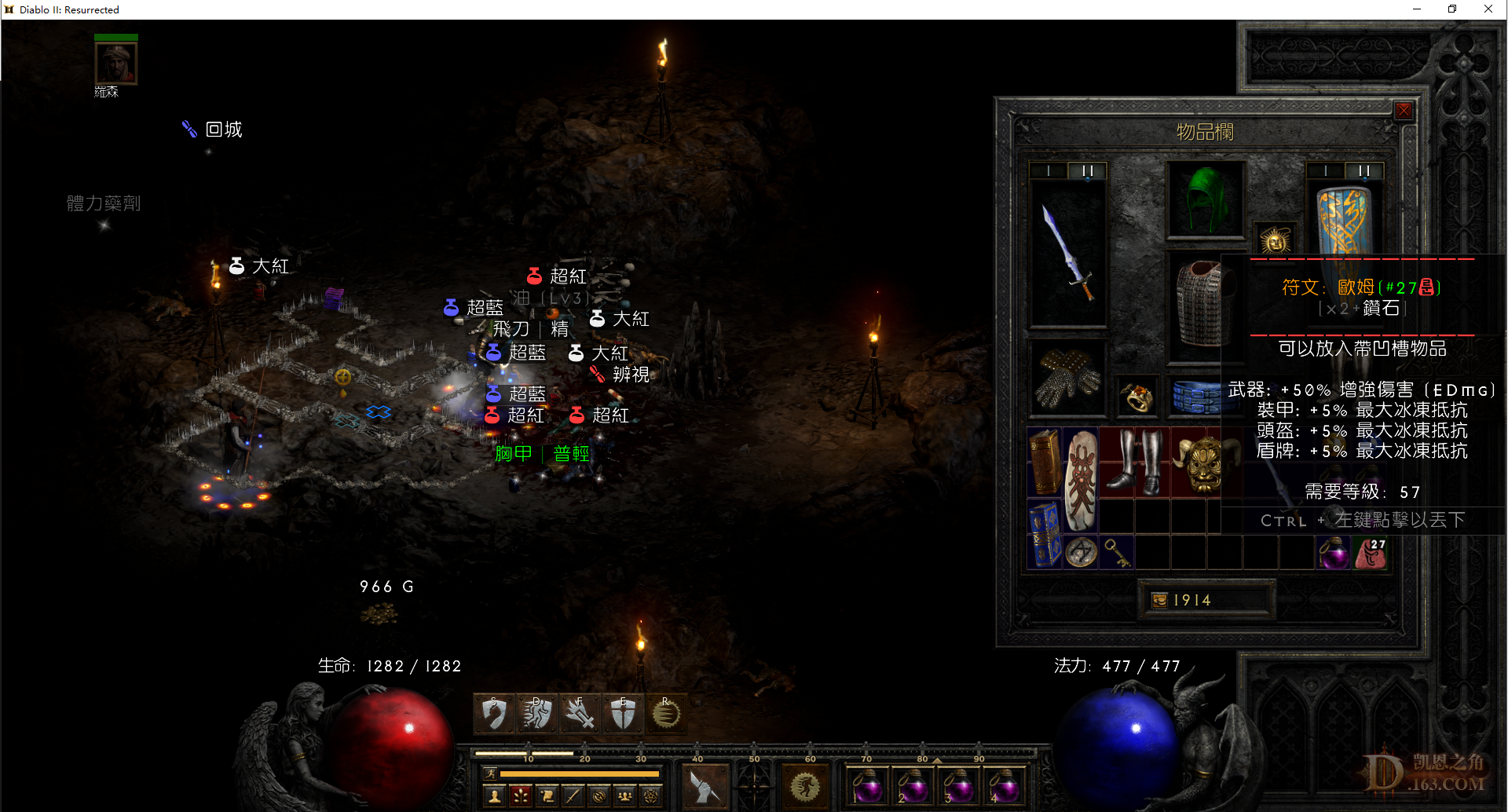//没登陆

 221~230：空。

33=0，32=0，31=0，30=0，29=0，28=0，27=3，26=0，25=0，24=2，格里风=0，次元=0，破隐=1，年纪=0，乔丹=2。
 231~240：空。 其实啊，我是来升级的，至于装备啊符文啊，无所谓了，有经验就行了。33=0，32=0，31=0，30=0，29=0，28=0，27=3，26=0，25=0，24=2，格里风=0，次元=0，破隐=1，年纪=0，乔丹=2。
 241~250：22#（不容易啊，第二个20+符文）。33=0，32=0，31=0，30=0，29=0，28=0，27=3，26=0，25=0，24=2，格里风=0，次元=0，破隐=1，年纪=0，乔丹=2。
 251~260：空。

33=0，32=0，31=0，30=0，29=0，28=0，27=3，26=0，25=0，24=2，格里风=0，次元=0，破隐=1，年纪=0，乔丹=2。
 261~270：4力量毒骨板，斗士的祸根。

33=0，32=0，31=0，30=0，29=0，28=0，27=3，26=0，25=0，24=2，格里风=0，次元=0，破隐=1，年纪=0，乔丹=2。
 271~280：27#！！！！（再创赛季新高），拿各，末日毁灭者。33=0，32=0，31=0，30=0，29=0，28=0，27=3，26=0，25=0，24=2，格里风=0，次元=0，破隐=1，年纪=0，乔丹=2。
 281~290：空。

33=0，32=0，31=0，30=0，29=0，28=0，27=3，26=0，25=0，24=2，格里风=0，次元=0，破隐=1，年纪=0，乔丹=2。
 顶顶顶顶顶顶

 291~300：空。

33=0，32=0，31=0，30=0，29=0，28=0，27=3，26=0，25=0，24=2，格里风=0，次元=0，破隐=1，年纪=0，乔丹=2。为楼主毅力点赞

 301~310：45抗库拉斯特之盾。

33=0，32=0，31=0，30=0，29=0，28=0，27=3，26=0，25=0，24=2，格里风=0，次元=0，破隐=1，年纪=0，乔丹=2。
 311~320：法师之拳，鸟弓*2。

33=0，32=0，31=0，30=0，29=0，28=0，27=3，26=0，25=0，24=2，格里风=0，次元=0，破隐=1，年纪=0，乔丹=2。
 321~330：玛那得的治疗。

33=0，32=0，31=0，30=0，29=0，28=0，27=3，26=0，25=0，24=2，格里风=0，次元=0，破隐=1，年纪=0，乔丹=2。
 331~340：35MF基德。

33=0，32=0，31=0，30=0，29=0，28=0，27=3，26=0，25=0，24=2，格里风=0，次元=0，破隐=1，年纪=0，乔丹=2。
 你这个可以啊